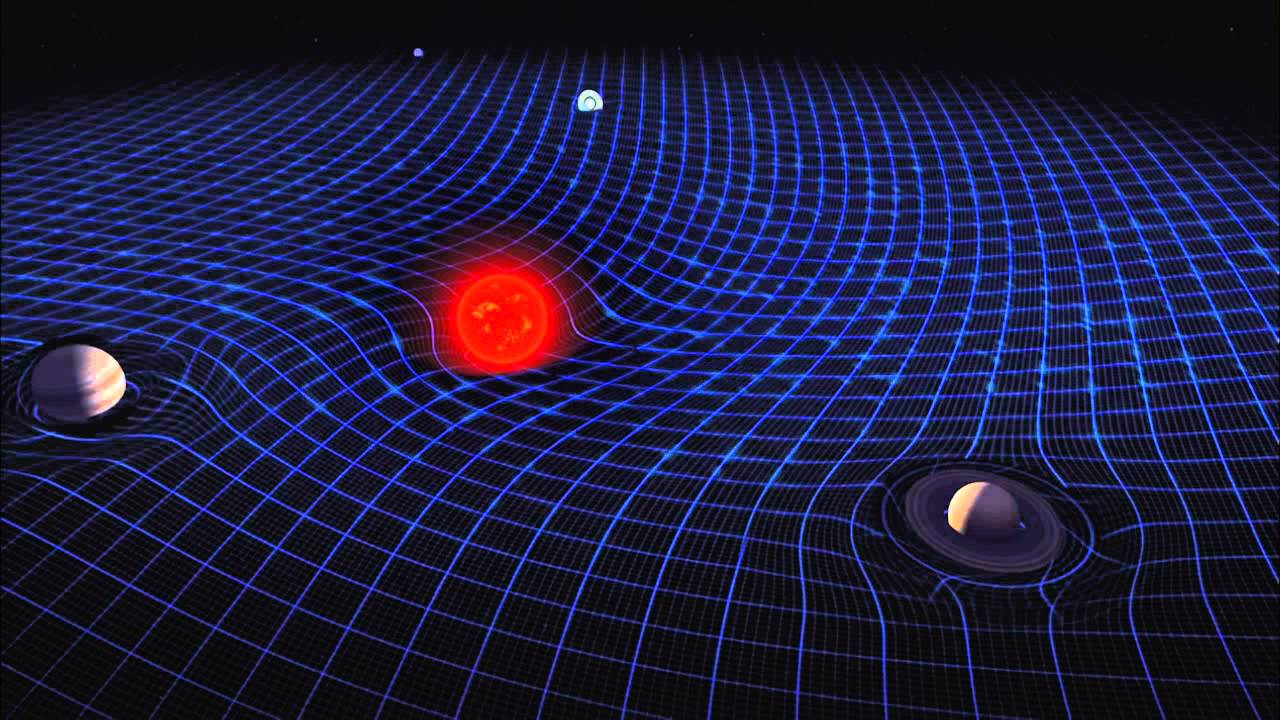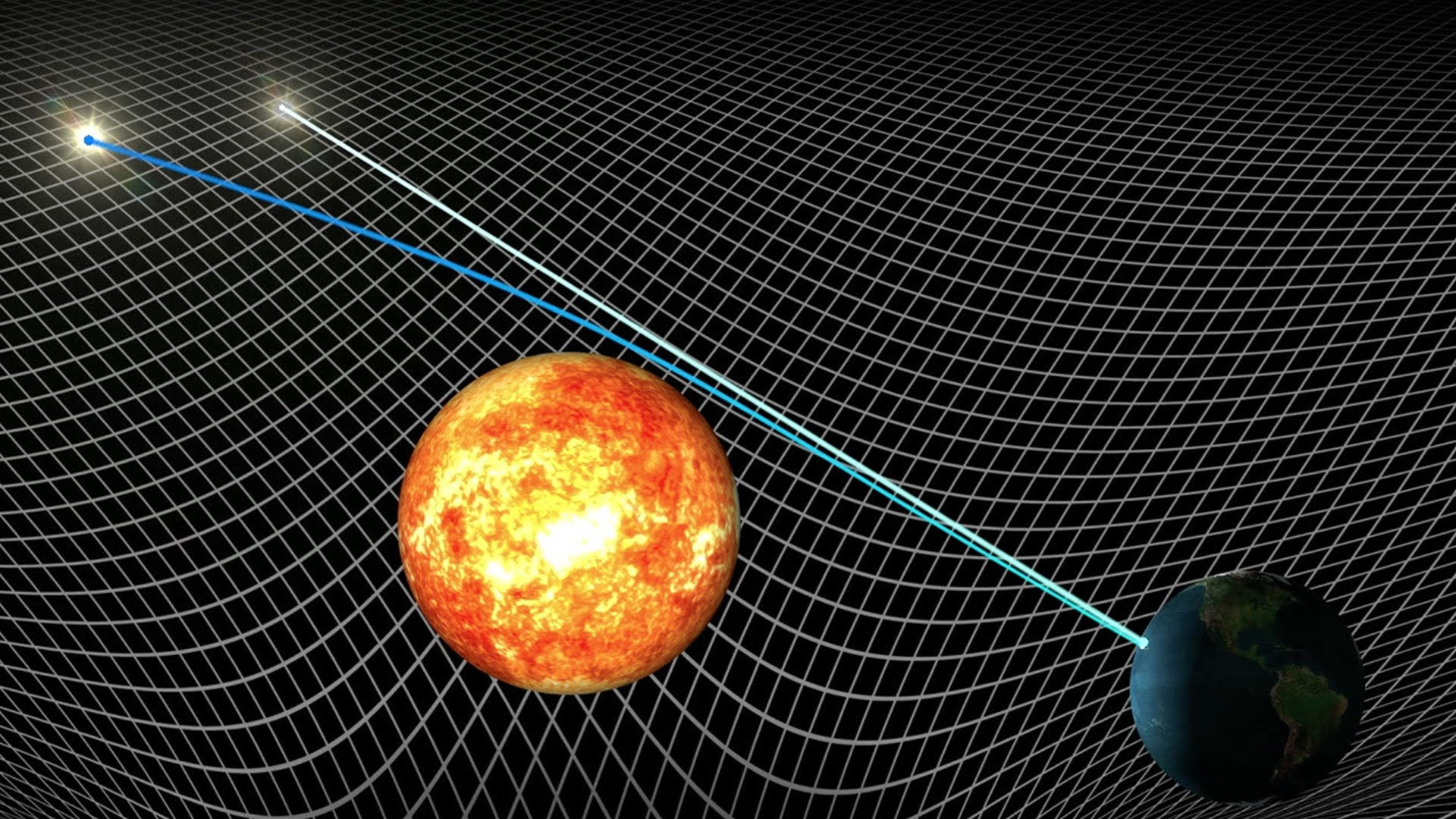# Gravity as a wave in space-time fabricWe know that every matter exerts a force on another matter in this universe known as gravitational force.But the question arises that what actually gravity is? How is it created? The whole universe is made up of energy, mass, space and time. A fabric called spacial fabric is the fundamental part of our universe. A sheet of space and time covers our whole universe. Its like a fabric. In the "flat" spacetime of special relativity, where gravity is absent, the laws of mechanics take on an especially simple form: As long as no external force is acting on an object, it will move on a straight line through space-time: at a constant velocity along a straight path. Now we add gravity to the situation, for instance by placing a massive sphere somewhere in space. In Newton's theory of gravity, this sphere will exert a force on all other masses around it. If we place a test particle in the vicinity, we see that its movement is deflected away from the usual straight space-time line - its path would be curved towards the sphere and it would become accelerated as it feels the sphere's attraction. In Einstein's geometric theory of gravity, the situation is described in a completely different way: A mass that we place in a region of space will lead to a distortion of space-time. Empty space-time is flat - it looks exactly like the space-time of special relativity. Space-time in the presence of masses is curved. In curved space-time, there are no straight lines - just as there are no straight lines on the surface of a sphere. Einstein's universe performs an ever-ongoing cosmic dance, with matter and space-time interacting So what is gravity, in Einstein's universe? Generally speaking, any distortion of space-time geometry. More precisely, there are two sides to gravity: In part, gravity is an observer artifact: it can be made to vanish by going into free fall. Most of the gravity that we experience here on earth when we see objects falling to the ground is of this type, which we might call "relative gravity". The remainder of gravity, "intrinsic gravity", if you will, manifests itself in tidal forces, and is associated with a specific property of geometry: The curvature of space-time.

Any matter in this universe curves slightly the space-time fabric, making a parallel line to converge at a point .suppose two parallel lines are moving in space-time fabric and there is a mass in front of them that curves to space-time fabric. These lines would be contracting towards that mass due to the curvature of special fabric. It seems that they undergo a force of attraction by that mass, i.e. gravity. but it is the curvature of space-time fabric that forced these line to converge towards mass .curvature depends on the mass of the matter the curvature of special fabric is directly proportional to the mass of the matter .it means that the curvature around the black hole is much large and →∞(infinity) .according to Einsteins theory of relativity.

1. It is not possible to tell that which object is moving and which is at rest.
2. The speed of light is same for all observers no matter what their perspective is.

From the following axioms we can prove following results: Imagine there are two observers in space one (newton) is at rest and another (Albert) is sitting in its spaceship and moving forward with velocity v and a reflector also moving with it as if connected with the imaginary rod. At any instant Albert emits a laser beam from its spaceship towards Newton, Itll be reflected back after reaching reflector towards the ship. From Albert's perspective light goes down and reflected in a straight line. But from Newtons perspective “v” shape is covered by it since the speed of light is constant always i.e. c time of Albert w.r.t Newton will slow and Newton clock will be faster than Albert. But from Albert perspective time will remain unchanged and this is known as time dilation. This results in length contraction, increase in mass, energy to be spent on K.E. will increase, time decreases.

In the above example when v i.e. speed of ship →c (speed of light)

From newton perspective:

1. Length of ship (l) →0

2. M (mass) →∞

3. Energy required →∞

4. T→0

From Albert perspective:

1. Length of universe→0

2. He will imagine a gravity field acting in opposite direction towards his spaceship making objects outside ship accelerating in opposite direction and his ship's engine are creating thrust to maintain equilibrium in the gravitation field. Hence time of newton t→0. “Therefore no matter can move more than c because as v →c then m→∞.”

Since gravity field can also change the way of electromagnetic wave and since c is constant even from the perspective of any observer, therefore, time dilates and changes on the effect of the curvature of space-time fabric.

Since black hole if infinitely dense and has a great mass due to this it curves space-time fabric infinitely, so that no one even light cannot escape out of it if we go closer to the black hole then due to infinite gravity time tends to 0.

# Gravity as a wave:

Since space fabric is flexible enough to curve by mass then it should also be able to ripple and vibrate. These can only be created by creating disturbance in space by some huge masses. Imagine rapidly orbiting neutron stars will create tremendous disturbance in space fabric and that disturbance will exhibit properties of wave-like motion and will move forward and is known by the gravitational wave. This wave will squeeze and stretch any mass in its path i.e. Earth also. LIGO team has experimentally proved that these waves exist by performing laser light test as follows: Imagine a right angle where an emitter is placed at the vertex of this angle and two reflectors one on the terminal side and one on the initial side are place at large and equal distances. There is one detector at the vertex of the angle near the emitter. When the laser is emitted in both directions simultaneously then they should be reflected back simultaneously at receiver. They are emitted such that when they will recombine during interference of waves, the crest will meet troughs and they will cancel each other and final photonic energy will remain zero. And detector will detect no photons. In case if the gravitational wave comes then the distance of both reflectors will vary and interference pattern of waves will shift which results in detection of photons (light).Since LIGO team has observed these waves, now we can explore our universe not only by electromagnetic waves but also by gravity waves. In future, these detections from unknown sources would help us to know and perceive information about that source.We can also study about masses of the black hole and their property. We can also find information about neutron stars and also study what happens during formation of the black hole.Note by Kunwar Pratap Singh
4 years, 2 months ago

This discussion board is a place to discuss our Daily Challenges and the math and science related to those challenges. Explanations are more than just a solution — they should explain the steps and thinking strategies that you used to obtain the solution. Comments should further the discussion of math and science.

When posting on Brilliant:

• Use the emojis to react to an explanation, whether you're congratulating a job well done , or just really confused .
• Ask specific questions about the challenge or the steps in somebody's explanation. Well-posed questions can add a lot to the discussion, but posting "I don't understand!" doesn't help anyone.
• Try to contribute something new to the discussion, whether it is an extension, generalization or other idea related to the challenge.
• Stay on topic — we're all here to learn more about math and science, not to hear about your favorite get-rich-quick scheme or current world events.

MarkdownAppears as
*italics* or _italics_ italics
**bold** or __bold__ bold
- bulleted- list
• bulleted
• list
1. numbered2. list
1. numbered
2. list
Note: you must add a full line of space before and after lists for them to show up correctly
paragraph 1paragraph 2

paragraph 1

paragraph 2

[example link](https://brilliant.org)example link
> This is a quote
This is a quote
    # I indented these lines
# 4 spaces, and now they show
# up as a code block.

print "hello world"
# I indented these lines
# 4 spaces, and now they show
# up as a code block.

print "hello world"
MathAppears as
Remember to wrap math in $$ ... $$ or $ ... $ to ensure proper formatting.
2 \times 3 $2 \times 3$
2^{34} $2^{34}$
a_{i-1} $a_{i-1}$
\frac{2}{3} $\frac{2}{3}$
\sqrt{2} $\sqrt{2}$
\sum_{i=1}^3 $\sum_{i=1}^3$
\sin \theta $\sin \theta$
\boxed{123}` $\boxed{123}$

## Comments

There are no comments in this discussion.

×

Problem Loading...

Note Loading...

Set Loading...